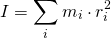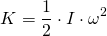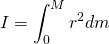# Physics - Classical Mechanics - Rotational Kinetic Energy & Moment of Inertia[Image 1]

## Introduction

Hey it's a me again @drifter1! Today we continue with Physics and more specifically the branch "Classical Mechanics" to get into Rotational Kinetic Energy and the so called Moment of Inertia. So, without further ado, let's get straight into it!

## Rotational Kinetic Energy

Any object that moves has some Kinetic Energy. For objects undergoing translational motion, that is based on the motion of their center of mass, we can easily calculate their (Translational) Kinetic Energy. But, what about objects rotating around a fixed axis of rotation or even objects that move from a point A to a point B, but "rolling" there? Rotational motion also has some kinetic energy that is defined as Rotational Kinetic Energy and it's calculation is exactly what we will be covering today. So, any object's Kinetic Energy is equal to the sum of Translational + Rotational Kinetic Energy. For some cases one of the two terms might be zero!

Thinking about the equation of "regular" kinetic energy: K = ½·m·Vcm2, we can recall that the kinetic energy is associated with the mass of the object m and also the velocity of the center of mass Vcm. Well, there's a small problem here! In rotational motion every point around the axis of rotation has a different velocity. So, let's think about some quantity that doesn't change. Of course angular velocity ω is the same for all points! You might remember that we can write the magnitude of the tangential velocity/speed as: vt = ω·r, where r is the distance of the point from the axis of rotation (radius).

Substituting this equation in the equation of kinetic energy we get:Of course this previous equation is only true for objects with points of the same radius (circle). What about the case of rigid rotating bodies? Well, then we just have to divide the body into a large number of smaller masses, where each mass has some distance to the axis of rotation, so that the total sum of the individual masses gives us the total mass of the object M. Each mass has some tangential speed, but the angular velocity is equal for all of them, and so the total rotational kinetic energy of the object becomes:## Moment of Inertia

Seeing how v is being replaced by ω in the translational kinetic energy equation, Physicists suggested to define a new rotational variable, to make the relationship between rotational and translational variables simpler. So, the sum in parentheses that we had in the previous equation is a counterpart of mass, when talking about rotational kinetic energy. This quantity is called Moment of Inertia and symbolized by the letter 'I'. It's S.I. unit is the kg·m2, based on the units of mass and distance. Mathematically this is written as:Substituting this quantity into the equation of Rotational Kinetic Energy we get:A much simpler equation! Based on this equation we understand that the kinetic energy of a rotating rigid body is directly proportional to the moment of inertia and square of angular velocity.

What remains now is how we can calculate the moment of inertia for common object shapes, so that we can simplify the process even more! Moment of inertia is described by a sum, but most commonly calculated by integral calculus. As an integral moment of inertia looks like this:Applying this integral for common shapes we end up with the following equations for common shapes:[Image2]

In examples we will mostly talk about such common shapes and combinations of them! I know that many people (including me) hate to apply complicated math in physics... :)

## RESOURCES:

### Images

1.2. http://hyperphysics.phy-astr.gsu.edu/hbase/mi.html

## Final words | Next up

This is actually it for today's post! Next time we will get into the Parallel Axis or Steiner Theorem, that's quite useful in calculations that have to do with Moments of Inertia...

See ya!Keep on drifting!

H2
H3
H4
3 columns
2 columns
1 column